Mathematics is an exact science where there is no margin for error no matter how minute. Mathematics is generally an abstract study of space, quantity, change, and structure. It is the science that resolves “what is true” or “what is false,” with the use of formulations and patterns. In cosmology, the use of math symbols is differentiated with numerology wherein the former deals with cosmic mathematical symbols while the latter deals with the study of numbers’ influence in one’s life.

## Infinity Symbol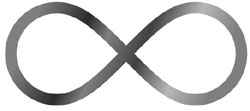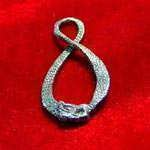What are some of most commonly used math symbols? The   (infinity sign) is highly popular math symbol. This could be attributed to the fact that “infinity” symbolizes that which has no end, one that lasts forever. John Wallis came up with the concept of infinite things in 1655. The symbol was a deviation from an ancient symbol depicting a dragon chasing and wanting to eat its very own tail. People tend to be drawn to the idea of infinity as it could relate to infinite love, wealth and all things right.

## Pi Sign

 The   (Pi) sign a math symbol that relates to a transcendental or mystical number which does not really equates to a quantifiable extent or quantity or size. Mathematically expressed, Pi is the ratio of a given circle’s circumference to its diameter. Though Pi is roughly equal to 3.1416 for the sake of solving mathematical problems, its real value is a series of numbers that go on infinitely as in 3.14159265358979323846… and on. Mystical Pi symbolized the universe as Pi and the universe produces non-repeating patterns, no sequences whatsoever to make them identical. It has been said that the Pi symbols are a sinister Masonic sign that only the most elite in the hierarchy of secret masons know what terror lies behind the Pi sign.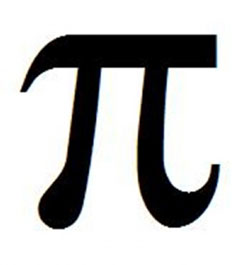## Sigma Sign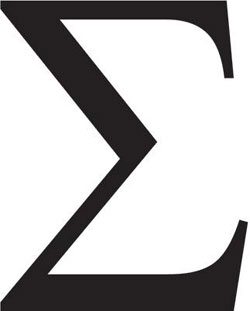The  (Sigma sign) is the 18th letter in the Greek alphabet. Mathematically, Sigma is a summation notation which basically means “sum it up”.  This math symbol was first used by Leonhard Euler, a Swiss mathematician, in representing the addition of a series of mathematical expressions.

## Golden Ratio

 Another popular math symbol is the Golden Ratio which is also called the divine proportion or golden section or golden mean. It deals with the numbers involved in the ratios of distances such as in the geometric shapes of a pentagram, pentagon, dodecahedron, and decagon. The divine proportion is symbolized by the figure Ø (Phi) or.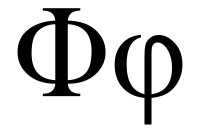## Fibonacci Spiral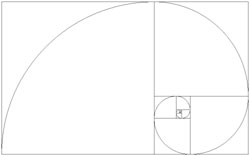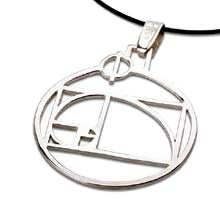In conjunction with the Golden Ratio is the Fibonacci spiral which is also identified as the golden spiral.  The golden ratio and golden spiral are geometric ratios found in numerous structures and patterns in the universe. It is the perfect ratio as exemplified by nature.  This math symbol intends to add tranquility and beauty to one’s life by being “one” with nature.

## Mobius Strip

 The Mobius is another common math symbol. Its single facet signifies the harmonious merger of the inner self and the outer world. Its lone facet symbolizes unity and oneness which is why the Mobius ring is now well-accepted wedding jewelry.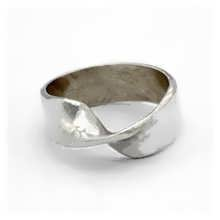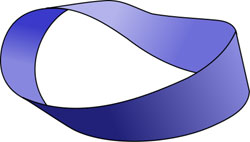## Delta

 Derived from the Phoenician ‘daleth’, Delta is the fourth letter in the Greek alphabet and has the value of 4 in the Greek numeral system. It is also used as a mathematical symbol that represents change or a finite difference in any changeable quantity. The upper case Delta is shaped as an isosceles triangle.